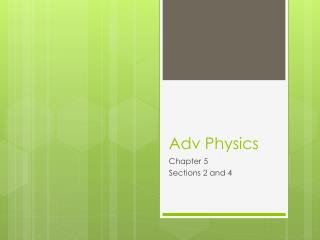DownloadDownload PresentationDownload Presentation- - - - - - - - - - - - - - - - - - - - - - - - - - - E N D - - - - - - - - - - - - - - - - - - - - - - - - - - -
##### Presentation Transcript

1. Adv Physics Chapter 5 Sections 2 and 4

2. Review • Work – force applied over a given distance W = F Δx [W] = Joules, J • Assumes the force is constant

3. Review • Energy – having the ability to do work • Kinetic Energy – energy due to motion KE = ½ mv2 [KE] = Joules, J

4. Review • Gravitational Potential Energy – energy an object has due to its height • Relative to a reference point GPE = mgh [GPE] = Joules, J

5. Review • Mechanical energy – energy due to motion and/or position • Total of KE and GPE

6. Elastic Potential Energy • Energy an object has due to its stretched or compressed position • Ex – spring, rubber band EPE = ½ kx2 k = spring constant x = amount spring is stretched or compressed [EPE] = Joules, J

7. Hooke’s Law • Whenever a spring is stretched or compressed, the spring tries to pull itself back to its equilibrium position • Restoring force – force that pulls a spring back to equilibrium • Greater the stretch or compression, the greater the restoring force F = -kx

8. Hooke’s Law F = -kx • F = restoring force • x = amount spring is compressed or stretched • Negative sign because the restoring force is always opposite the direction of displacement, x • k = spring constant a number that indicates the stiffness of the spring (bigger the k, the stiffer the spring) [k] = N/m

9. Sample Problem • If a mass of 0.55 kg attached to a vertical spring stretches the spring 2 cm from its original equilibrium position, what is the spring constant? • What if the spring is replaced with a spring that stretches 36 cm from its equilibrium position. What is the spring constant in this case?

10. Sample Problem • A slingshot consists of a light leather cup attached between two rubber bands. If it takes a force of 32 N to stretch the bands 1.2 cm, what is the equivalent spring constant of the rubber bands? • How much force is required to pull the cup 3 cm from its equilibrium position?

11. Elastic Potential Energy and Work • Energy a spring has because its been stretched or compressed EPE = ½ kx2 • To give a spring EPE requires work • Can’t use W = FΔx since the force changes with distance W = ΔEPE = EPEf – EPEi = ½ kxf2 – ½ kxi2

12. Sample Problem • A spring with a force constant of 5.2 N/m has a relaxed length of 2.45 m. When a mass is attached to the end of the spring and allowed to come to rest, the vertical length of the spring is 3. 57 m. Calculate the elastic potential energy stored in the spring.

13. Sample Problem • A spring with a force constant of 50 N/m is to be stretched from 0 to 20 cm. (a) The work required to stretch the spring from 10 to 20 cm is (1) more than, (2) the same as, (3) less than that required to stretch it from 0 to 10 cm. (b) Compute the two work values.

14. Sample Problem • A particular spring has a force constant of 2500 N/m. (a) How much work is done in stretching the relaxed spring by 6 cm? (b) How much more work is done in stretching the spring an additional 2 cm?

15. Sample Problem • A 1.5 kg mass is placed on the end of a spring that has a spring constant of 175 N/m. The mass-spring system rests on a frictionless incline that is at an angle of 30 degrees from the horizontal. The system is eased into its equilibrium position, where it stays. • (a) Determine the change in elastic potential energy of the system. • (b) Determine the system’s change in gravitational potential energy.